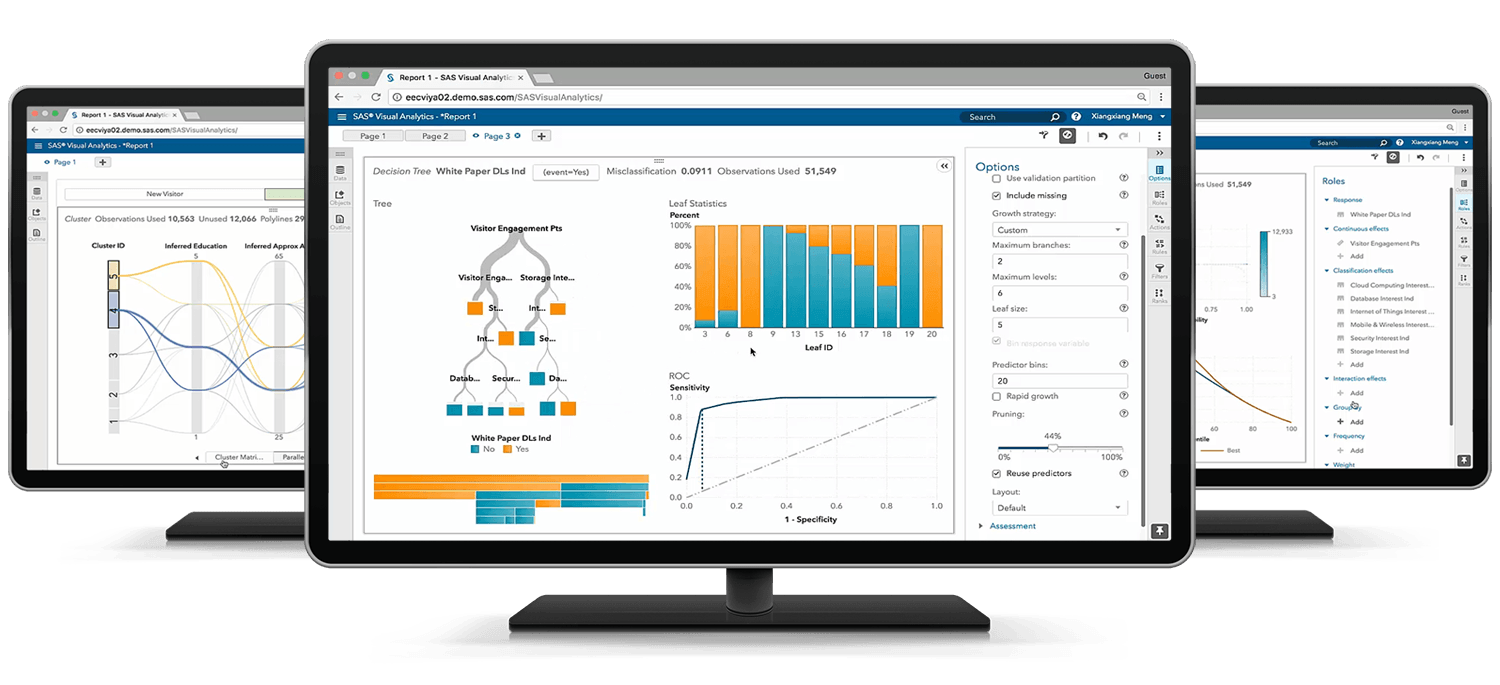SAS Certified Specialist:Natural Language Processing and Computer Vision Using SAS Viya 3.4

Exam Content Guide

Below we provide a list of the objectives that will be tested on the exam.
For more specific details about each objective download the complete exam content guide.• Import documents for analysis
• Create and explore a project in SAS® Visual Text Analytics
• Load and prepare image data

Identifying Text Patterns Using Natural Language Processing Techniques (42%)

• Use the Concepts and Text Parsing Nodes to extract Terms and Concepts
• Write concept rules
• Use the Topics Node to extract machine-generated topics
• Use rules to identify documents belonging to specific categories
• Write category rules
• Use a Recurrent Neural Network (RNN) to recognize patterns

Identifying Image Patterns Using Computer Vision Techniques (38%)

• Use convolutional layers in a Convolutional Neural Network (CNN)
• Use padding in a Convolutional Neural Network (CNN)
• Use pooling in a Convolutional Neural Network (CNN)
• Use fully connected layers in a Convolutional Neural Network (CNN)
• Use output layers in a Convolutional Neural Network (CNN)
• Tune the Hyperparameters of a Convolutional Neural Network (CNN)
• Score new image data
• Explain the impact of various architectural designs
• Use regularization techniques